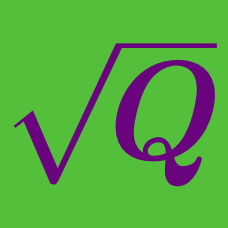Algebra

# Radical Expressions and Equations: Level 3 Challenges

$\LARGE \sqrt{3} - \sqrt{5}$

Which one of these choices is equivalent to the expression above?

$\large \frac{4}{\big(\sqrt{5} + 1\big)\big(\sqrt{5} + 1\big)\big(\sqrt{5} + 1\big)\big(\sqrt{5} + 1\big)}$

If the above expression is equal to $x$, find the value of $(x+1)^{64}$.

$\sqrt{3+\sqrt{3}+\sqrt{2+\sqrt{3}+\sqrt{7+\sqrt{48}}}} = a + \sqrt{b}$

If $a$ and $b$ are positive integers, find $a + b$.

$\large \sqrt{4+\sqrt{4-\sqrt{ 4+\sqrt{4-\sqrt{ 4+\sqrt{4-\dots}}}}}}$

What is the closed form of the above expression?

The equation $x + \sqrt{x-2} = 4$ has $\text{\_\_\_\_\_\_\_\_\_\_}.$

×